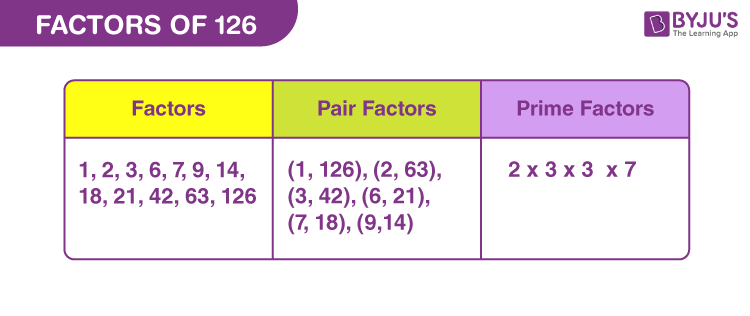# Factors of 126

In Mathematics, factors of 126 are the natural numbers, also called divisors, by which the original number is evenly divisible. 126 is an even composite number, hence it will have factors, more than two.

If the result of multiplication of two natural numbers is 126, then such numbers are the factors of 126. The factors could be common to other numbers as well. For example, if 2 is a factor of all the even numbers such as 4, 16, 28, 60, 100, 126, etc. Hence, a number can be a factor of more than one natural number. But 1 is the factor of all the numbers.## How to Find the Factors of 126?

A factor divides the original number, uniformly. Therefore, we can find the factors of 126 by dividing it by other natural numbers.

• 126 ÷ 1 = 126
• 126 ÷ 2 = 63
• 126 ÷ 3 = 42
• 126 ÷ 6 = 21
• 126 ÷ 7 = 18
• 126 ÷ 9 = 14
• 126 ÷ 14 = 9
• 126 ÷ 18 = 7
• 126 ÷ 21 = 6
• 126 ÷ 42 = 3
• 126 ÷ 63 = 2
• 126 ÷ 126 = 1

Therefore, the factors of 126: 1, 2, 3, 6, 7, 9, 14, 18, 21, 42, 63 and 126.

## Pair Factors of 126

The factors when multiplied in pairs to get the original number, are called the pair factors.

• 1 × 126 = 126
• 2 × 63 = 126
• 3 × 42 = 126
• 6 × 21 = 126
• 7 × 18 = 126
• 9 × 14 = 126

Therefore, the pair factors of 126 are (1, 126), (2, 63), (3, 42), (6, 21), (7, 18) and (9, 14).

The negative pair factors can be written as the product of two negative numbers that result in 126.

• -1 × -126 = 126
• -2 × -63 = 126
• -3 × -42 = 126
• -6 × -21 = 126
• -7 × -18 = 126
• -9 × -14 = 126

Therefore, the negative pair factors are (-1, -126), (-2, -63), (-3, -42), (-6, -21), (-7, -18) and (-9, -14).

## Prime Factorisation of 126

Since 126 is a composite number, therefore, we can determine the prime factors of 126 that can exactly divide the original number. The prime factorisation of 126 results in:

 126 ÷ 2 = 63 63 ÷ 3 = 21 21 ÷ 3 = 7 7 ÷ 7 = 1

Therefore, the prime factor of 126 is 2, 3 and 7.

 Prime factorisation of 126 = 2 × 3 × 3 × 7 Exponential form = 21 × 32 × 71

## Solved Examples

Q.1: What should be multiplied by 21 to get 126?

Solution: Suppose, x is the number to be multiplied by 21 to get 126.

So, x × 21 = 126

x = 126/21

x = 6

Therefore, 6 should be multiplied by 21 to get 126.

Q.2: What is the sum of factors of 126?

Solution: The factors of number 126 are 1, 2, 3, 6, 7, 9, 14, 18, 21, 42, 63, 126.

Sum of all the factors = 1 + 2 + 3 + 6 + 7 + 9 + 14 + 18 + 21 + 42 + 63 + 126

Sum = 312

Therefore, 312 is the required sum.

Q.3: What is the greatest common factor of 120 and 126?

Solution: The factors of 120 and 126, respectively, are:

120 → 1, 2, 3, 4, 5, 6, 8, 10, 12, 15, 20, 24, 30, 40, 60, 120

126 → 1, 2, 3, 6, 7, 9, 14, 18, 21, 42, 63, 126

Therefore, the greatest common factor of 120 and 126 is:

GCF (120, 126) = 6

### Practice Questions

1. What are the even divisors of 126?
2. Find the common factors of 126 and 132.
3. What is the GCF of 98 and 126?
4. What are the prime factors of 126?

## Frequently Asked Questions on Factors of 126

### What are the factors of 126?

The factors of 126 are 1, 2, 3, 6, 7, 9, 14, 18, 21, 42, 63 and 126.

### What are the multiples of 126?

The first ten multiples of 126 are 126, 252, 378, 504, 630, 756, 882, 1008, 1134, 1260.

### What is the prime factor form of 126?

The prime factor form of 126 is:
126 = 2 x 3 x 3 x 7 = 2 x 32 x 7

### Is 126 a perfect square?

126 is not a perfect square.

### Is 126 a composite number or prime number?

126 is a composite number.